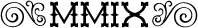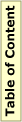## MMIX Bugmmix-20110831

### Bug Reported

Initial: 27/3/2012

Martin Ruckert

### Description

The interval counter rI measures the elapsed time in clock cycles but is decremented in mmix-sim by 1 for each instruction. Further, the filter features of rU are not implemented in mmix-sim.

### Details

mmix-doc specifies in section 40:
The interval Counter rI decreases by 1 on every "clock pulse" of the MMIX processor.

mmix-doc specifies in section 50:
Each operation will be assumed to take an integer number of υ, where υ (pronounced "oops") is a unit that represents the clock cycle time in a pipelined implementation.

...

The usage counter rU consists of three fields (up, um, uc), called the usage pattern up, the usage mask um, and the usage count uc. The most significant byte of rU is the usage pattern; the next most significant byte is the usage mask; and the remaining 48 bits are the usage count. Whenever an instruction whose OP ∧ um=up has been executed, the value of uc increases by 1 (mod 2 u48).

This specification is implemented in mmix-pipe.

mmix-sim specifies in section 1:
All instructions take a fixed amount of time, given by the rough erstimates stated in the MMIX documentation. For example, MUL takes 10υ, LDB takes μ + υ ... The simulated clock increases by 232 for each μ and 1 for each υ. But the interval counter rI decreases by 1 for each instruction, and the usage counter rU increases by 1 for each instruction.

This is not in agreement with the specification of mmix-doc and creates a systematic difference between mmix-sim and mmix-pipe.

While the simulated time measured in υ is available to the interactive user of mmix-sim by measn of the sclock variable, it is not available to the running program. The running program has access only to rI and rU. The usage counter correctly counts instructions but the filter mechanism is not implemented as specified. It can be used as a replacement for the present rI if the number of instructions is required. Without a correct implementation of rI, the running program can not accurately reflect on its running time.

### Proposed patch

Two patches effect the documentation.
@@ -52,3 +52,3 @@
-$2^{32}$ for each~$\mu$ and 1~for each~$\upsilon$. But the interval
-counter~rI decreases by~1 for each instruction, and the usage
-counter~rU increases by~1 for each instruction.
+$2^{32}$ for each~$\mu$ and 1~for each~$\upsilon$. The interval
+counter~rI decreases by~1 for each~$\upsilon$, and the usage
+count field of~rU may increase by~1~(mod~$2^{48}$) for each instruction.

@@ -189 +189 @@
-cause a break in simulation after 250 instructions have been executed.
+cause a break in simulation after $250\upsilon$ have elapsed.

One patch is necessary to handle the extra two cycles of a bad guess.
@@ -2114 +2115,5 @@
- else bad_guesses++, sclock.l+=2; /* penalty is $2\upsilon$ for bad guess */
+ else {
+   bad_guesses++, sclock.l+=2; /* penalty is $2\upsilon$ for bad guess */
+   if (g[rI].h==0 && g[rI].l<=2) tracing=breakpoint=true;
+   g[rI]=incr(g[rI],-2);
+ }

And one patch fixes the code to update the clocks. The actual code is largely taken from mmix-pipe.
 @@ -2646,8 +2651,14 @@
@=
-if (g[rU].l || g[rU].h || !resuming) {
+if (sclock.l || sclock.h || !resuming) {
sclock.h+=info[op].mems; /* clock goes up by $2^{32}$ for each $\mu$ */
-  sclock=incr(sclock,info[op].oops); /* clock goes up by 1 for each $\upsilon$ */
-  g[rU]=incr(g[rU],1); /* usage counter counts total instructions simulated */
-  g[rI]=incr(g[rI],-1); /* interval timer counts down by 1 only */
+  sclock.l+=info[op].oops; /* clock goes up by 1 for each $\upsilon$ */
+  if ((!(loc.h&sign_bit) || (g[rU].h&0x8000)) &&
+      (op&(g[rU].h>>16))==g[rU].h>>24) {
+      g[rU].l++;@+ if (g[rU].l==0) {
+        g[rU].h++;@+ if ((g[rU].h&0x7fff)==0) g[rU].h-=0x8000;@+
+      }
+    } /* usage counter counts total instructions simulated */
+  g[rI]=incr(g[rI],-1); /* interval timer goes down by 1 only */
if (g[rI].l==0 && g[rI].h==0) tracing=breakpoint=true;
+  g[rI]=incr(g[rI],-(info[op].oops-1)); /* interval timer goes down by 1 for ea
ch $\upsilon$ */
}


### Discussion

The patch as given above is now part of the sources.

Please help to keep this site up to date! If you want to point out important material or projects that are not listed here, if you find errors or want to suggest improvements, please send email to